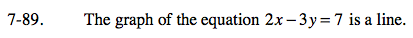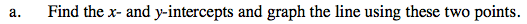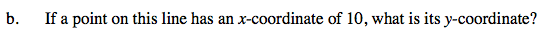### Home > AC > Chapter 7 > Lesson 7.3.1 > Problem7-89

7-89.
1. The graph of the equation 2x − 3y = 7 is a line. Homework Help ✎

1. Find the x- and y-intercepts and graph the line using these two points.

2. If a point on this line has an x-coordinate of 10, what is its y-coordinate?Substitute x = 10 into the original equation and solve for y.

$\text{If }x =10, \ y = \frac{13}{3}.$